# SAT II Math I : Midpoint Formula

## Example Questions

2 Next →

### Example Question #11 : Midpoint Formula

A line is connected by points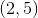and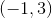on a graph.  What is the midpoint?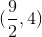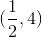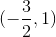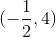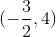Explanation:

Write the midpoint formula.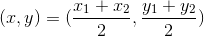Let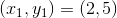and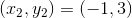.

Substitute the given points.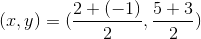Simplify the coordinate.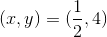The answer is:### Example Question #12 : Midpoint Formula

Find the midpoint between (2,8) and (8,6).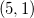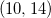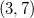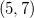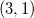Explanation:

The midpoint formula says that: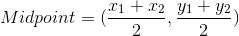Given (2,8) and (8,6);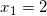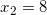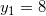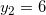Plug the values into the formula and reduce: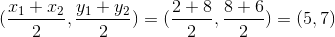### Example Question #13 : Midpoint Formula

Find the midpoint between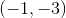and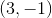.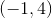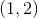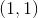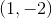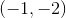Explanation:

Write the formula for the midpoint.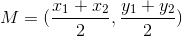Substitute the point into the formula.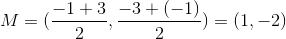The answer is:2 Next →